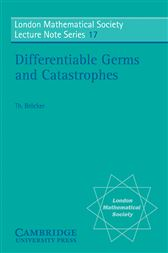# Differentiable Germs and Catastrophes

## Series: London Mathematical Society Lecture Note Series (No. 17)US\$38.00
US\$33.99
These notes give a fairly elementary introduction to the local theory of differentiable mappings. Sard's Theorem and the Preparation Theorem of Malgrange and Mather are the basic tools and these are proved first. There follows a number of illustrations including: the local part of Whitney's Theorem on mappings of the plane into the plane, quadratic differentials, the Instability Theorem of Thom, one of Mather's theorems on finite determinacy and a glimpse of the theory of Toujeron. The later part of the book develops Mather's theory of unfoldings of singularities. Its application to Catastrophe theory is explained and the Elementary Catastrophes are illustrated by many pictures. The book is suitable as a text for courses to graduates and advanced undergraduates but may also be of interest to mathematical biologists and economists.